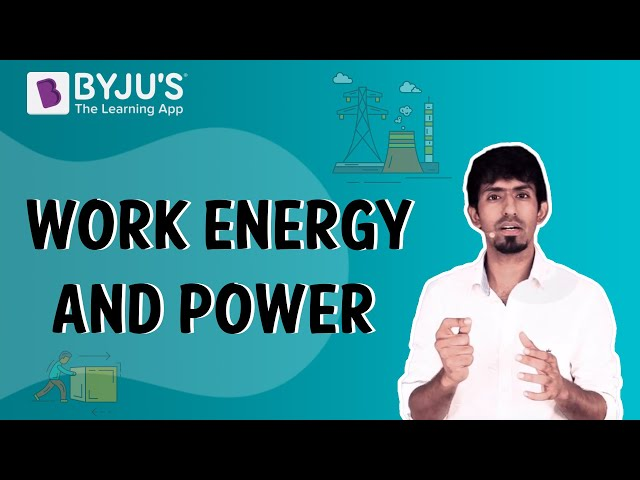# Class 11 Physics Chapter 6 Work Energy and Power MCQs

Class 11 Physics Chapter 6 Work, Energy and Power MCQs are provided here with answers. These questions are designed as per the latest CBSE syllabus and NCERT curriculum. Solving these chapter-wise MCQs will help students to score good marks in the final exam. Work, Energy and Power Class 11 physics MCQs are prepared for a better understanding of the concept. It allows students to test their knowledge and answering skills in the given time frame.

## MCQs on Class 11 Chapter 6 Work, Energy and Power

Check the multiple-choice questions for the 11th Class Physics Work, Energy and Power chapter. Each MCQ will have four options here, out of which only one is correct. Students have to pick the correct option and check the answer provided here.

Download Chapter 6 Work, Energy and Power MCQs PDF by clicking on the button below.

1. The rate of doing work is called ——————-

1. Force
2. Acceleration
3. Power
4. Displacement

Explanation: Power is defined as the rate of doing work.

2. Which is the type of collision in which both the linear momentum and the kinetic energy of the system remain conserved?

1. Inelastic Collision
2. Elastic Collision
3. Destructive collision
4. None of the options

Explanation: In an elastic collision, the linear momentum and the kinetic energy of the system remain conserved.

3. Collision between marble balls is which type of collision?

1. Inelastic Collision
2. Elastic Collision
3. Destructive collision
4. None of the options

Explanation: Collision between marble balls is an example of elastic collision.

4. The energy possessed by the body by virtue of its motion is known as?

1. Chemical energy
2. Thermal energy
3. Potential energy
4. Kinetic energy

Explanation: The energy possessed by a body by virtue of its motion is known as its kinetic energy. It is given by the formula,

$$\begin{array}{l}KE=\frac{1}{2}mv^{2}\end{array}$$

5. Find the potential energy stored in a ball of mass 5 kg placed at a height of 3 m above the ground.

1. 121.20 J
2. 147.15 J
3. 227.31 J
4. 182.21 J

Explanation: m = 5 kg, h = 3 m, g = 9.81 m/s-2

We know that, Potential energy = mgh

= 5 * 9.81 * 3 = 147.15 J

6. What is the power utilised when work of 1000 J is done in 2 seconds?

1. 100 W
2. 200 W
3. 20 W
4. 500 W

Explanation: W=1000J, t=2 seconds

Power=work/time = 1000/2 = 500 W

7. State true or false: According to Equivalence of Mass and Energy, it states that mass and energy are NOT interconvertible.

1. True
2. False

Explanation: According to Equivalence of Mass and Energy put forth by Einstein, it states that mass and energy are interconvertible.

$$\begin{array}{l}E=mc^{2}\end{array}$$

8. Which one has higher kinetic energy? Both light and heavy bodies have equal momenta.

1. Heavy body
2. Light body
3. Both
4. None of the options

Explanation: Since, the momenta of the heavy body and light body are same, thus the velocity of light body is greater than that of heavy body. Also, kinetic energy is proportional to the square of velocity. Hence, the lighter body has greater kinetic energy.

9. An electric heater of rating 1000 W is used for 5 hrs per day for 20 days. What is the electrical energy utilized?

1. 100 kWh
2. 200 kWh
3. 120 kWh
4. 500 kWh

Explanation: The power of the electric heater is 1000 W, and the time period is 20×5 = 100 hr.

Electrical energy = Power × Time

Electrical energy = 1000 × 100 = 100000 Wh

Electrical energy = 100 kWh

10. A ball moves in a frictionless inclined table without slipping. The work done by the table surface on the ball is

1. Negative
2. Zero
3. Positive
4. None of the options